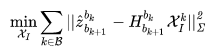## 从零手写VIO——（六）VINS 初始化和 VIO 系统

519
0
2020年10月8日 17时50分

IMU 传感器模型：

IMU 预积分：

## VINS 鲁棒初始化

① 旋转外参数未知，则先估计旋转外参数.

② 利用旋转约束估计陀螺仪 bias.

③ 利用平移约束估计重力方向，速度，以及尺度初始值.

④ 对重力向量进一步优化.

⑤ 求解世界坐标系和初始相机坐标系之间的旋转矩阵，并将轨迹对齐到世界坐标系（可以用也就是相机坐标系的重力加速度，与对比，求算出之间的旋转关系）.

1. Zhenfei, Yang, Shaojie,等. Monocular Visual–Inertial State Estimation With Online Initialization and Camera–IMU Extrinsic Calibration[J]. IEEE Transactions on Automation Science & Engineering, 2017.

2. Qin T , Shen S . Robust initialization of monocular visual-inertial estimation on aerial robots[C]// IEEE/RSJ International Conference on Intelligent Robots & Systems. IEEE, 2017:4225-4232.就可以展开为：

bool InitialEXRotation::CalibrationExRotation(vector<pair<Vector3d, Vector3d>> corres, Quaterniond delta_q_imu, Matrix3d &calib_ric_result)
{
frame_count++;
Rc.push_back(solveRelativeR(corres));
Rimu.push_back(delta_q_imu.toRotationMatrix());
Rc_g.push_back(ric.inverse() * delta_q_imu * ric);

Eigen::MatrixXd A(frame_count * 4, 4);
A.setZero();
int sum_ok = 0;
for (int i = 1; i <= frame_count; i++)
{
Quaterniond r1(Rc[i]);
Quaterniond r2(Rc_g[i]);

double angular_distance = 180 / M_PI * r1.angularDistance(r2);
//ROS_DEBUG("%d %f", i, angular_distance);

double huber = angular_distance > 5.0 ? 5.0 / angular_distance : 1.0;
++sum_ok;
Matrix4d L, R;

double w = Quaterniond(Rc[i]).w();
Vector3d q = Quaterniond(Rc[i]).vec();
L.block<3, 3>(0, 0) = w * Matrix3d::Identity() + Utility::skewSymmetric(q);
L.block<3, 1>(0, 3) = q;
L.block<1, 3>(3, 0) = -q.transpose();
L(3, 3) = w;

Quaterniond R_ij(Rimu[i]);
w = R_ij.w();
q = R_ij.vec();
R.block<3, 3>(0, 0) = w * Matrix3d::Identity() - Utility::skewSymmetric(q);
R.block<3, 1>(0, 3) = q;
R.block<1, 3>(3, 0) = -q.transpose();
R(3, 3) = w;

A.block<4, 4>((i - 1) * 4, 0) = huber * (L - R);
}

JacobiSVD<MatrixXd> svd(A, ComputeFullU | ComputeFullV);
Matrix<double, 4, 1> x = svd.matrixV().col(3);
Quaterniond estimated_R(x);
ric = estimated_R.toRotationMatrix().inverse();
//cout << svd.singularValues().transpose() << endl;
//cout << ric << endl;
Vector3d ric_cov;
ric_cov = svd.singularValues().tail<3>();
if (frame_count >= WINDOW_SIZE && ric_cov(1) > 0.25)
{
calib_ric_result = ric;
return true;
}
else
return false;
}是相机测得的 k 到 k+1 的变换，是 imu 测得的，这里用视觉修正 imu。

void solveGyroscopeBias(map<double, ImageFrame> &all_image_frame, Vector3d* Bgs)
{
Matrix3d A;
Vector3d b;
Vector3d delta_bg;
A.setZero();
b.setZero();
map<double, ImageFrame>::iterator frame_i;
map<double, ImageFrame>::iterator frame_j;
for (frame_i = all_image_frame.begin(); next(frame_i) != all_image_frame.end(); frame_i++)
{
frame_j = next(frame_i);
MatrixXd tmp_A(3, 3);
tmp_A.setZero();
VectorXd tmp_b(3);
tmp_b.setZero();
Eigen::Quaterniond q_ij(frame_i->second.R.transpose() * frame_j->second.R);
tmp_A = frame_j->second.pre_integration->jacobian.template block<3, 3>(O_R, O_BG);
tmp_b = 2 * (frame_j->second.pre_integration->delta_q.inverse() * q_ij).vec();
A += tmp_A.transpose() * tmp_A;
b += tmp_A.transpose() * tmp_b;

}
delta_bg = A.ldlt().solve(b);
// ROS_WARN_STREAM("gyroscope bias initial calibration " << delta_bg.transpose());

for (int i = 0; i <= WINDOW_SIZE; i++)
Bgs[i] += delta_bg;

for (frame_i = all_image_frame.begin(); next(frame_i) != all_image_frame.end( ); frame_i++)
{
frame_j = next(frame_i);
frame_j->second.pre_integration->repropagate(Vector3d::Zero(), Bgs);
}
}而不是的原因是的这个负号。(18) bool LinearAlignment(map &all_image_frame, Vector3d &g, VectorXd &x) { int all_frame_count = all_image_frame.size(); int n_state = all_frame_count * 3 + 3 + 1; MatrixXd A{n_state, n_state}; A.setZero(); VectorXd b{n_state}; b.setZero(); map::iterator frame_i; map::iterator frame_j; int i = 0; for (frame_i = all_image_frame.begin(); next(frame_i) != all_image_frame.end(); frame_i++, i++) { frame_j = next(frame_i); MatrixXd tmp_A(6, 10); tmp_A.setZero(); VectorXd tmp_b(6); tmp_b.setZero(); double dt = frame_j->second.pre_integration->sum_dt; tmp_A.block<3, 3>(0, 0) = -dt * Matrix3d::Identity(); tmp_A.block<3, 3>(0, 6) = frame_i->second.R.transpose() * dt * dt / 2 * Matrix3d::Identity(); tmp_A.block<3, 1>(0, 9) = frame_i->second.R.transpose() * (frame_j->second.T - frame_i->second.T) / 100.0; tmp_b.block<3, 1>(0, 0) = frame_j->second.pre_integration->delta_p + frame_i->second.R.transpose() * frame_j->second.R * TIC - TIC; //cout << "delta_p " << frame_j->second.pre_integration->delta_p.transpose() << endl; tmp_A.block<3, 3>(3, 0) = -Matrix3d::Identity(); tmp_A.block<3, 3>(3, 3) = frame_i->second.R.transpose() * frame_j->second.R; tmp_A.block<3, 3>(3, 6) = frame_i->second.R.transpose() * dt * Matrix3d::Identity(); tmp_b.block<3, 1>(3, 0) = frame_j->second.pre_integration->delta_v; //cout << "delta_v " << frame_j->second.pre_integration->delta_v.transpose() << endl; Matrix cov_inv = Matrix::Zero(); //cov.block<6, 6>(0, 0) = IMU_cov[i + 1]; //MatrixXd cov_inv = cov.inverse(); cov_inv.setIdentity(); MatrixXd r_A = tmp_A.transpose() * cov_inv * tmp_A; VectorXd r_b = tmp_A.transpose() * cov_inv * tmp_b; A.block<6, 6>(i * 3, i * 3) += r_A.topLeftCorner<6, 6>(); b.segment<6>(i * 3) += r_b.head<6>(); A.bottomRightCorner<4, 4>() += r_A.bottomRightCorner<4, 4>(); b.tail<4>() += r_b.tail<4>(); A.block<6, 4>(i * 3, n_state - 4) += r_A.topRightCorner<6, 4>(); A.block<4, 6>(n_state - 4, i * 3) += r_A.bottomLeftCorner<4, 6>(); } A = A * 1000.0; b = b * 1000.0; x = A.ldlt().solve(b); double s = x(n_state - 1) / 100.0; // ROS_DEBUG("estimated scale: %f", s); g = x.segment<3>(n_state - 4); // ROS_DEBUG_STREAM(" result g " << g.norm() << " " << g.transpose()); if(fabs(g.norm() - G.norm()) > 1.0 || s < 0) { return false; } RefineGravity(all_image_frame, g, x); s = (x.tail<1>())(0) / 100.0; (x.tail<1>())(0) = s; // ROS_DEBUG_STREAM(" refine " << g.norm() << " " << g.transpose()); if(s < 0.0 ) return false; else return true; }

VINS-Mono中参数化方法：三维向量自由度为 2，采用球面坐标参数化：可以构建一个新的坐标系，表示空间内任意一个三维向量。是上一个步骤中估计出的重力向量，其中，是待优化变量。

• 找到到世界坐标系的旋转矩阵• 把相机坐标系下的变量旋转到世界坐标系下。
• 把相机平移和特征点尺度恢复到米制单位。

VINS 系统优化的状态变量为：